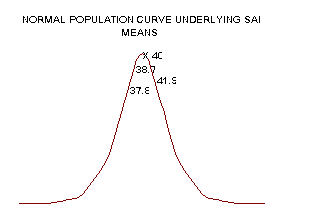previous lesson

Nature of statistical inference

POPULATION AND SAMPLING

A. Population vs. samples

1. Population = the whole thing being studied (universe).

2. Sample = a portion of the population.

Based upon what is found from the sample, one can infer (generalize) about the population. It is crucial that the sample is representative and random. There are statistics available to test inference (see chart).

B. Random procedures

1. Definition: Every unit in the whole population has an equal chance of being selected.

Example: Population = 100,000 marbles, each with a person's name on it. One marble is drawn, the name is read and returned to the population (in order to keep sample random). The chances of picking the first marble was 1/100,000. If the first marble had not been returned to the population, the second marble would have 1/99,999 chance.

2. Table of random numbers: Can be used in any systematic way, as long as consistency is maintained. Yet, even if a sample is drawn exactly "by the book", it may not be representative of the population.

3. Chance fluctuation: Representative samples may not necessarily be representative because it is not the population.

Examples:

population = 5000 white marbles and 12 red

sample = 10 white marbles -- this is not representative.

population = 5000 white and 5000 red marbles

sample = 10 white marbles -- this is not representative.

DESCRIPTION VS. INFERENCE

A. Description: The only way to be sure if a sample is representative is to compare the central tendency and dispersion of the sample to the entire population. The problem with this comparison is the whole population may not have been measured and the researcher is depending on the sample to gain information.

B. Inference: Take a sample and then make a general statement about the population. Certain statistics may increase confidence in generalizing, but there will always be a probability of being wrong. Increased sample size decreases the chances of an unrepresentative sample.

LOGIC OF INFERENCE

A. Inference Problem Examples: Mean, Association, Differences

1. Means: If the samples are drawn randomly, then even if the populations distribution is not normal the distribution of the sample means will be a normal distribution.Example:
Population:

x = 40 years
Samples:

x1 = 38.7 years

x2 = 41.9 years

x3 = 37.8 years, etc.

2. Association
Example:

population: G (gamma) = 0 : no relationship

samples: G1 = + 0.5 G2 = -0.1 etc.

A frequency polygon of the sample gamma results will approach a normal distribution as the sample size increases, even though the measured variable itself is not normally distributed in the whole population.

B. Null Hypothesis

1. Ha = research hypothesis = statement trying to prove.

2. Ho = null hypothesis = logical opposite of hypothesis. This usually means a hypothesis of no difference or no relationship. (By disproving Ho , Ha is "proven".)

C. Probability levels: used for all inferential statistics.

p > .05 cannot safely generalize

5/100 p = .05 minimum probability level for generalizing to the population

p < .05

(1/100) p = .01

p < .01

Where: p = probability of being wrong when generalizing from the sample to the whole population.